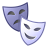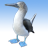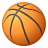# How do I normalize raster data?

20258
18
01-26-2012 01:30 PMNew Contributor II
I want to combine different raster layers, but need the data to be measured at the same numerical scale. Therefore, I would like to normalize all of the different layers so I can add them together, and then renormalize them.

I am not very good with statistics in general, and am unsure of how to even normalize a data set. I know there are multiple equations to do this, but don't know which one is right for me.

But if we were to use the equation:

Z = X - u / std

Where, Z - the normalized value;
u - mean; and,
std - standard deviation;

How would I calculate this in a raster?

I have tried using "Calculate Statistics" (Data Management Tools) because I assumed it would calculate mean and standard deviation for me, but I don't know where the output goes, as I can't choose that in the window. Also, the information on what this tool actually calculates is pretty limited in desktop help.

I have thought about using Focal Statistics to calculate mean and standard deviation individually, but I run into the problem of what scale to use - I want it to include the entire raster in the calculation. This tool would give me different outputs for mean and standard deviation, which I could then put into the raster calculator to solve the normalization equation.

Can someone please let me know if using Focal Statistics is the best way to go about this? Am I even using the right equation to normalize my data?

Any input is GREATLY appreciated!!
Tags (3)
18 RepliesOccasional Contributor III
I have a toolbox available that has a normalize options in the Statistics > transformations tool.

http://conserveonline.org/workspaces/emt/documents/arcgis-geomorphometrics-toolbox/view.htmlNew Contributor II
Thanks! I will give it a shot and let you know if its what I am looking for!New Contributor
I have a toolbox available that has a normalize options in the Statistics > transformations tool.

http://conserveonline.org/workspaces/emt/documents/arcgis-geomorphometrics-toolbox/view.html

Hi Jeffrey,

This tool sounds great.

Is the tool only available for v10?  Unfortunately I'm still on v9.3.1 and won't be moved to v10 for a while.

WendyOccasional Contributor III
Sorry, I should have been clear that the toolbox was only compatible with ArcGIS 10. You are on the right track with calculate statistics. Make sure you set the skip value to 1, then add the raster to ArcMap (or navigate to it in ArcCatalog) and right click on it and select properties. In the layer properties box select the source tab and scroll down to the bottom and you will see the statistics section and can retrieve the min, max, mean and stdv (can copy and paste the values). You can then use the values to perform a normalization on your raster in the raster calculator (Arctoolbox > Spatial Analyst Tools > Map Algebra > Raster Calculator) with the following syntax.

(x - mean) / stdv
where; x is your raster, mean and stdv are the real values of the respective statistical moments.

The above formula from your original post does not transform to a standard variable space. This transformation is intended to scale the mean to 0 and stdv to 1 while maintaining the shape of the original distribution. The resulting data range is dictated by the range in your original data. This formula, in effect, makes the negative and positive bounds symmetrical (e.g., -1 to 1). If the original data distribution is non-normal the results can be unexpected. If you just want your data in the same scale and it is all positive, you could just perform a "row standardization" by dividing your raster by its max value to (mostly) return a range of 0-1. Here is a method that accounts for negative values and also reliably returns a range of 0-1. ( x - min(x) ) / ( max(x) - min(x) )

If you have access to R, the behavior of these three transformations can be readily observed given a normal and skewed distribution. Note that you can plot the distribution of any of the resulting transformations and it does not change shape, just range. Here is the code (just copy and paste).

############################
#  Based on a non-normal distribution
############################
x <-runif(100,1,100)
summary(x)
plot(density(x))

x1 <- ( x - mean(x) ) / sd(x)
summary(x1)

x2 <- x / max(x)
summary(x2)

x3 <- ( x - min(x) ) / ( max(x) - min(x) )
summary(x3)

# When distribution has negative values (note that the regular row standardization [x/max(x)] does not scale correctly)
x <- -10
x2 <- x / max(x)
x3 <- ( x - min(x) ) / ( max(x) - min(x) )
summary(x2);summary(x3)

############################
# Based on a non-normal distribution
############################
x <- rweibull(1e5,1.5,33)
summary(x)
plot(density(x))

x1 <- ( x - mean(x)) / sd(x)
summary(x1)

x2 <- x / max(x)
summary(x2)

x3 <- ( x - min(x) ) / ( max(x) - min(x) )
summary(x3)New Contributor
Dear all,
Geomorphometry and Gradient Metrics Toolbox (GGMT) is a powerful tool.
Thanks for Jeffrey�??s works!

For to normalize raster data, I tried three ways:
1) Using raster calculator in ArcGIS, (x - mean) / stdv --> ( x - min(x) ) / ( max(x) - min(x) )
2) Using GGMT, Statistics --> transformations --> normalize
3) Using GGMT, Statistics --> transformations --> standardize --> stretch 0~1
The new layers transformed through the three different ways are the same results.

My question is following:
Before running Species Distribution Models such as MaxEnt, I don't know whether to normalize, standardize, and rescale (0~1) the environmental raster layers is a necessary step.
If that is necessary!
How should I do that in GGMT?Occasional Contributor III
The decision to transform your data is dependent on the type of model that you are using and the results of an exploratory analysis of your data. If you were using a parametric method such as OLS (Ordinary Least Square Regression) or ENFA (Ecological Niche Factor Analysis), I would certainly transform my covariates. However, the most powerful models for species distribution modeling (MaxEnt, Random Forests, etc...) are nonparametric and, as such, do not have IID or distributional assumptions. Because of this no data transformations are necessary.New Contributor
Hi Jeffrey,

When I want to compare different methods (including MaxEnt and ENFA)
whether it is a good idea to calculate raster
using (x - mean) / stdv --> (x - min(x)) / (max(x) - min(x))
for all environmental variables before running species distribution models.

What is the difference between �??normalize�?� and �??standardize�?� in Geomorphometry and Gradient Metrics Toolbox.
Their algorithms are�?�New Contributor
Once again,
thanks superman jeff evans!
jen hooperNew Contributor
Hello, friend.

Remember that, for standardizing data, you assume that this data is in a normal distribution. If the data is not in a normal distribution, it is advisable to use percentile rank instead of normalization. A good explanation about the theory and about how to do it in SPSS are in these links:

http://www.psychstat.missouristate.edu/introbook/sbk14m.htm